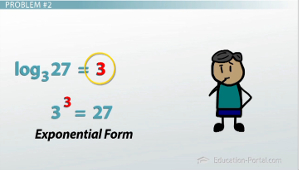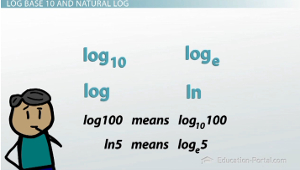# How to Evaluate Logarithms

An error occurred trying to load this video.

Try refreshing the page, or contact customer support.

Coming up next: Logarithmic Properties

### You're on a roll. Keep up the good work!

Replay
Your next lesson will play in 10 seconds
• 0:10 Review of Logarithmic Form
• 1:39 Logarithm Problem Examples
• 3:27 Log Base 10 and Natural Log
• 4:35 Change of Base Formula
• 6:05 Lesson Summary

Want to watch this again later?

Timeline
Autoplay
Autoplay
Speed

#### Recommended Lessons and Courses for You

Lesson Transcript
Instructor: Ori Gold
Using this lesson, you can get practice evaluating logarithms, as well as learn some of the shortcuts behind writing and estimating them. You can also learn how to use your calculator to evaluate logarithms, and learn about a concept called the change of base theory.

## Quick Review of Logarithmic Form

We learned that logarithms are the inverse operation of exponentials, and they give us a way to solve exponential equations. When we were trying to figure out how far into the future it will be before there are 200 billion cell phones, we were stuck with the equation 2x=34,359,738,368. That's where the log came in, because the answer to our question, 'what exponent turns 2 into 34, 359,738,368?' is exactly what log2 (34,359,738,368) is. We decided that the log2 of 34,359,738,368 was 35, because 235=34,359,738,368. What you see are two different ways of writing the exact same thing; I could write this equation in logarithmic form, which is logb (y) = x, or I could write it in exponential form, which is bx=y. And both of these forms are just two different ways of saying the same thing.Now, a lot of students get freaked out when they see a log, because it's new, and they're not used to it, and think that it means to multiply log with 5, or to raise 5 to the 25th power… but neither of these are actually true. The little 5 beneath the log is a 'subscript' and goes with the log. Every log is going to have one of these, and it tells us which type of log we are doing.

## Problem #1

In this example, we are taking the log base 5 of 25 - so we're taking the log5 of this number. Therefore, we are being asked, 5 to what power equals 25? The answer is, of course, 2. So the answer to our log is simply 2, because 52 = 25.

## Problem #3

Another example: How about log9 (1) = ? What exponent turns 9 into 1? Well, you have to remember the 0 exponent property - remember that anything to the 0 power gives you 1, so 0 is the exponent that turns 9 into 1, so the answer to this one is just 0.

## Problem #4Or, finally, what about log2 (1/8) = ? For this one you're going to have to remember the negative exponent property. Negative exponents give us fractions, and because I have to turn 2 into 8, that is the 3rd power, because 2 * 2 * 2 = 8, so 2(-3) = 1/8, and therefore the answer to log2 (1/8) is, simply, -3.

To unlock this lesson you must be a Study.com Member.

### Register to view this lesson

Are you a student or a teacher?

### Unlock Your Education

#### See for yourself why 30 million people use Study.com

##### Become a Study.com member and start learning now.
Back
What teachers are saying about Study.com

### Earning College Credit

Did you know… We have over 160 college courses that prepare you to earn credit by exam that is accepted by over 1,500 colleges and universities. You can test out of the first two years of college and save thousands off your degree. Anyone can earn credit-by-exam regardless of age or education level.